Support Center

# How do I insert mathematical expressions or equations?

## Write beautiful math equations with the Insert Math tool. For more advanced equations, you can even write LaTeX code directly.

You can draw simple math using the Draw tool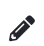, or type math using the Text tool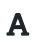.

For more advanced mathematical equations or expressions, you can use the Insert Math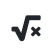tool.

Use the Editor tab to write math with an editor, or the LaTeX code tab to insert equations with LaTeX. The editor supports many operations, such as inserting an exponent by typing the ^ character, or creating a fraction by typing the / character. For more advanced use cases, see the LaTeX examples below to use with the LaTeX code tab.

You can also use an online LaTeX equation editor to produce LaTeX code to insert via the Insert Math tool's LaTeX code tab. There is also a brief introduction to LaTeX symbols available here.

### LaTeX examples

 Equation LaTeX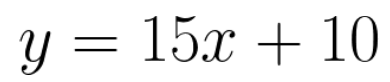y = 15x + 10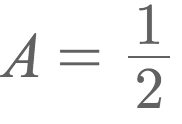Use \frac{dividend}{divisor} to display fractions. A = \frac{1}{2}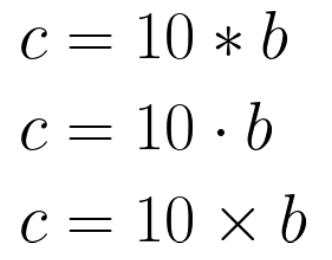LaTeX supports multiple different multiplication symbols. c = 10*b c = 10 \cdot b c = 10 \times b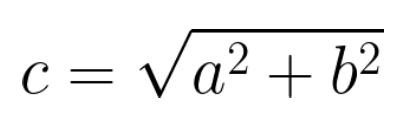c = \sqrt{a^2 + b^2}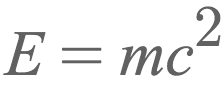E = mc^2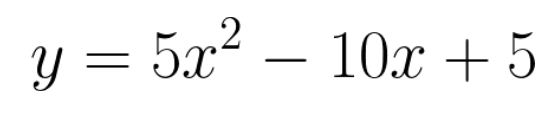y = 5x^2 - 10x + 5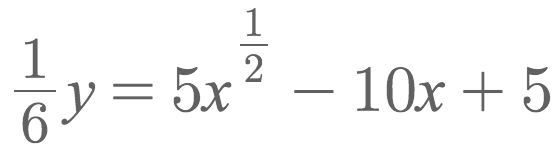\frac{1}{6}y = 5x^{\frac{1}{2}} - 10x + 5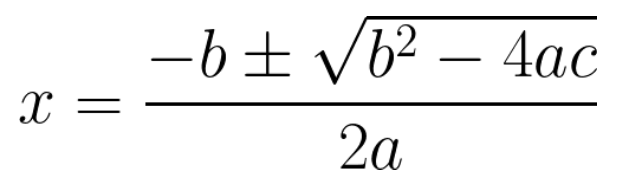x = \frac{-b \pm \sqrt{b^2-4ac}}{2a}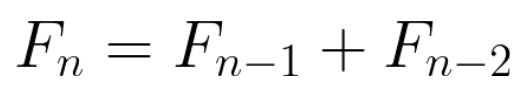F_n = F_{n - 1} + F_{n - 2}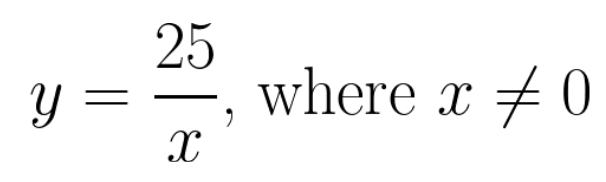Use \text{ } to write text inside the equation. y = \frac{25}{x} \text{, where } x \neq 0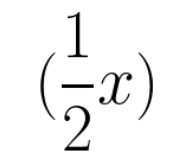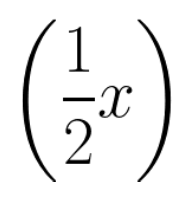Use \left( and \right) for parentheses which cover the entire height of an expression. (\frac{1}{2}x) \left(\frac{1}{2}x\right)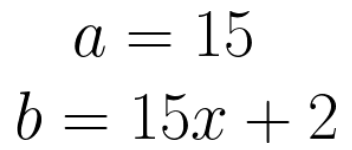Use two backwards slashes (\\) to place a line break. a = 15 \\b = 15x + 2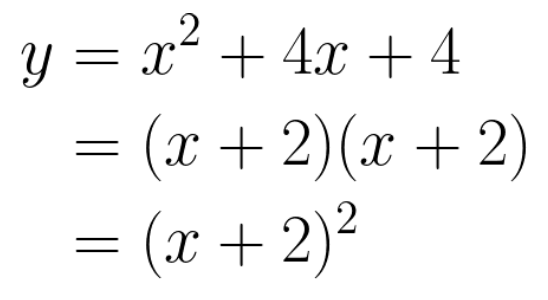Use the align* block to align multi-line equations. Use the ampersand symbol (&) on each line to indicate which part should be aligned. \begin{align*}y &= x^2 + 4x + 4 \\&= (x+2)(x+2) \\&= (x+2)^2\end{align*}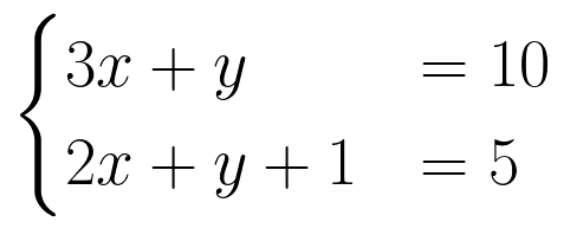Use the cases block to style a simultaneous equation. Combine with the align* block to align each equation with the & symbol. \begin{align*}\begin{cases}3x + y &= 10 \\2x + y + 1 &= 5\end{cases}\end{align*}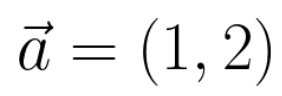\vec{a} = (1,2)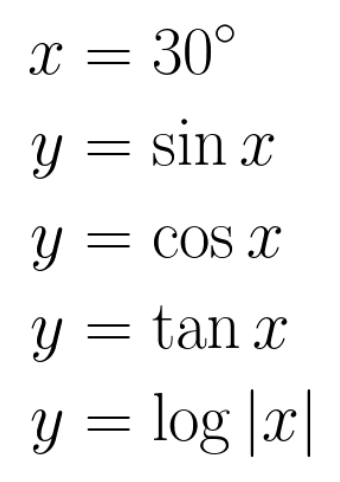x = 30^\circ  y = \sin x y = \cos x y = \tan x y = \log |x|

### List of symbols

For a list of LaTeX symbols to insert please see List of LaTeX mathematical symbols or try the Detexify symbol recognition tool.